#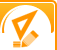Mean, Median and Range## Purpose

To determine whether the mean, median and range of the height of students will differ from one grade to the next.

The mean of a set of numbers is the average – when all the numbers are added and divided by the number of numbers in the set, this is the mean. The median number is the number that numerically, is in the middle of all the numbers. The range is the difference between the smallest number in the set and the largest.

## Required materials

• Volunteer students in one grade and volunteer students in the next grade up
• A yardstick or other means to measure a student’s height
• Journal or Logbook
• Calculator

Several hours.

## Step-By-Step Procedure

• 1. Gather the student volunteers from the lowest grade and measure their heights. Record the data in the logbook.
• 2. Gather the student volunteers from the next grade up and measure their heights. Make sure to record the data in your journal or logbook.
• 3. Calculate the mean, median and range for the heights of students in the lowest grade as well as the mean median and range for the heights of students in the next grade up.

## Note

This project is excellent for discovering how mean, median and range can be helpful when applied to situations that present themselves in real life.

## Observation

Once the calculations are complete, compare the numbers. What is the mean of the heights of the students in the lowest grade? What is the median? What is the range? What are the mean, median and range of the heights of the students in the next grade up? How do the numbers differ, if they differ at all? Answering these types of questions will help you analyze how the mean, median and range of the heights of the students compare and how these results can be applied to every day life.

## Result

You will most likely find that that the mean, median and range of the heights of the students increase with grade level and age. How much do the numbers increase by? What do you think would happen if you measured the mean, median and range of the heights of students another grade higher? How can knowing how to calculate these numbers be helpful in real life situations?

Take a moment to visit our table of Periodic Elements page where you can get an in-depth view of all the elements, complete with the industry first side-by-side element comparisons!

 Message: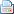Print this page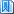Bookmark this pageHide all projects# All Projects List

•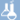Chemistry
•Biology
•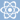Physics
•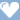Medicine & Health
•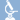Biochemistry
•Environmental science
•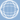Earth science
•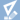Mathematics
•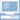Computer science